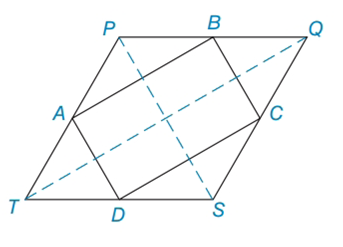Chapter 4.3, Problem 25EElementary Geometry For College St...

7th Edition
Alexander + 2 others
ISBN: 9781337614085

Solutions

Chapter
SectionElementary Geometry For College St...

7th Edition
Alexander + 2 others
ISBN: 9781337614085
Textbook Problem

In Exercises 25 and 26, supply the missing statements and reasons. Given: Quadrilateral PQST with midpoints A, B, C, and D of the sides Prove: ABCD is a ▱PROOF Statements Reasons 1. Quadrilateral PQST with midpoints A, B, C, and D of the sides 1. ? 2. Draw T Q ¯ 2. Through two points, there is one line 3. A B ¯ | | T Q ¯  in  △ T P Q 3. The line joining the midpoints of two sides of a triangle is | | to the third side 4. D C ¯ | | T Q ¯  in  △ T S Q 4. ? 5. A B ¯ | | D C ¯ 5. ? 6. Draw P S ¯ 6. ? 7. A D ¯ | | P S ¯  in  △ T S P 7. ? 8. B C ¯ | | P S ¯  in  △ P S Q 8. ? 9. A D ¯ | | B C ¯ 9. ? 10. ? 10. If both pairs of opposite sides of a quadrilateral are | | , the quadrilateral is a ▱

To determine

To supply:

The missing statements and reasons and prove ABCD is a .

Explanation

ASA Rule for triangle congruency:

Calculation:

The inner parallelogram creates four separate triangles. We must prove that the triangles opposite each other a congruent.

 PROOF Statements Reasons 1. Quadrilateral PQST with midpoints A, B, C, and D of the sides 1. Given 2. Draw TQ¯ 2. Through two points, there is one line 3. AB¯||TQ¯ in △TPQ 3. The line joining the midpoints of two sides of a triangle is || to the third side 4. DC¯||TQ¯ in △TSQ 4. The line joining the midpoints of two sides of a triangle is || to the third side. By ASA (Angle Side Angle), the opposite triangles are congruent. 5. AB¯||DC¯ 5

Still sussing out bartleby?

Check out a sample textbook solution.

See a sample solution

The Solution to Your Study Problems

Bartleby provides explanations to thousands of textbook problems written by our experts, many with advanced degrees!

Get Started

In Exercises 75-98, perform the indicated operations and/or simplify each expression. 94. (x2 1)(2x2 x2(2x)

Applied Calculus for the Managerial, Life, and Social Sciences: A Brief Approach

Evaluate the integral. 01(1+r)3dr

Calculus (MindTap Course List)

Prove each identity. seccos()=sin2cos

Trigonometry (MindTap Course List)

Find the sum of the series. 28. n=11n(n+3)

Single Variable Calculus: Early Transcendentals